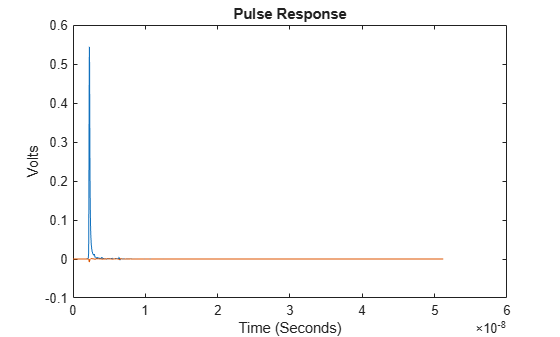# impulse2pulse

Pulse response from impulse response

## Syntax

``P = impulse2pulse(I,N,dt)``

## Description

example

````P = impulse2pulse(I,N,dt)` converts the impulse response `I` to a pulse response `P`, given the number of samples per symbol `N` and the uniform sampling interval `dt`.```

## Examples

collapse all

Load the impulse response column matrix from a file.

`load('PulseResponseReflective100ps.mat');`

Calculate the pulse response from the impulse response.

`pulse = impulse2pulse(impulse,SamplesPerSymbol,dt);`

Plot the pulse response.

``` figure plot(t,pulse) xlabel('Time (Seconds)') ylabel('Volts') title('Pulse Response')```## Input Arguments

collapse all

Input impulse response, specified as a column matrix. The first column contains the primary impulse response and the subsequent columns (if any) contain the crosstalk impulse responses.

Data Types: `double`

Number of samples per symbol, specified as a positive integer scalar.

Data Types: `double`

Uniform timestep of the waveform, specified as a real positive scalar in seconds.

Data Types: `double`

## Output Arguments

collapse all

Converted pulse response, returned as a column matrix. The first column contains the primary pulse response and the subsequent columns (if any) contain the crosstalk pulse responses.

Data Types: `double`

## Version History

Introduced in R2020a